# Chem 108 Class Lab Week 11 Pick a

• Slides: 31Chem 108: Class/ Lab Week 11 Pick a vial and a plastic dropper Using the vial number, sign-in on the Lab roster 1) TODAY: Fluid Exchange (Handout) Due Next Lab 2) Acid-Base Equilibrium Experiment (Handout) Due & signed Today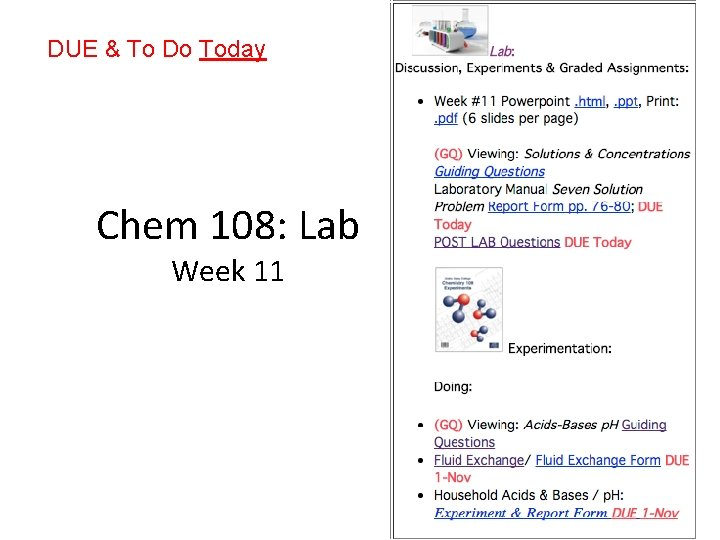DUE & To Do Today Chem 108: Lab Week 11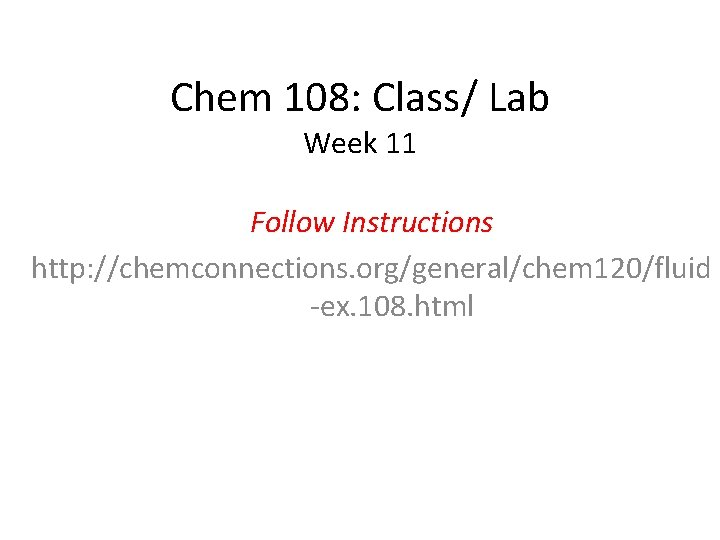Chem 108: Class/ Lab Week 11 Follow Instructions http: //chemconnections. org/general/chem 120/fluid -ex. 108. htmlAcid-Base Indicators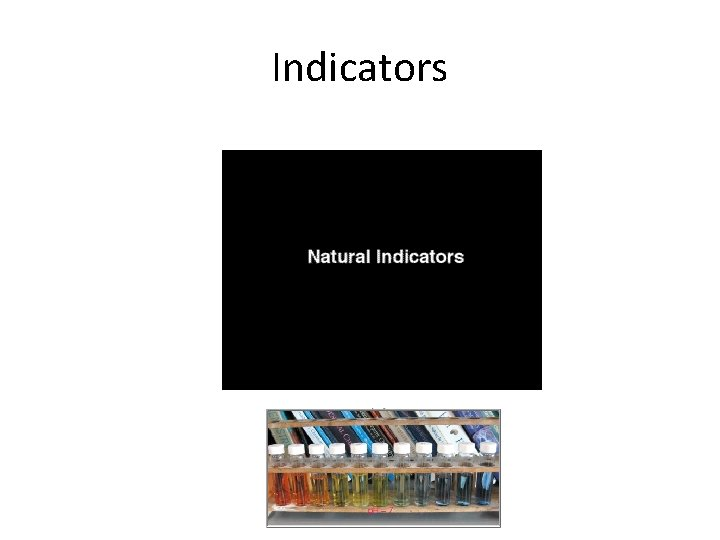IndicatorsAcid-Base IndicatorsWater as an Acid and a Base Self-ionization http: //chemconnections. org/general/movies/Kw. Activity. swfWater is neutral It is an Acid and a Base What is equilibrium? ¥H 2 O + H 2 O H 3 O+ + OH acid 1 base 2 conj acid 2 conj base 1 ¥Kw = 1 10 14 at 25°C ¥Kw = [H 3 O+ ][OH ] = [1 10 7 M]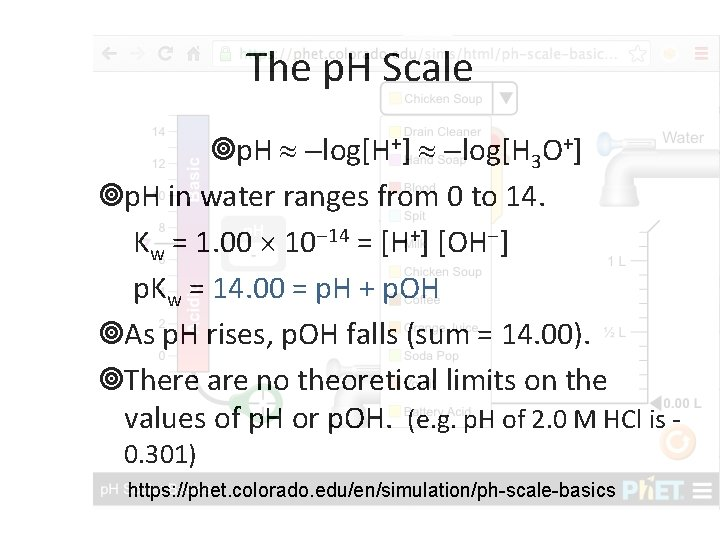The p. H Scale ¥p. H log[H+] log[H 3 O+] ¥p. H in water ranges from 0 to 14. Kw = 1. 00 10 14 = [H+] [OH ] p. Kw = 14. 00 = p. H + p. OH ¥As p. H rises, p. OH falls (sum = 14. 00). ¥There are no theoretical limits on the values of p. H or p. OH. (e. g. p. H of 2. 0 M HCl is 0. 301) https: //phet. colorado. edu/en/simulation/ph-scale-basicsAcid-Base Strengths p. H [indicator paper & p. H meter] https: //phet. colorado. edu/en/simulation/ph-scale Strong Acid: Strong Base: Weak Acid: Weak Base: http: //www. chemconnections. org/general/chem 108/Acids-Bases%20 Guide. html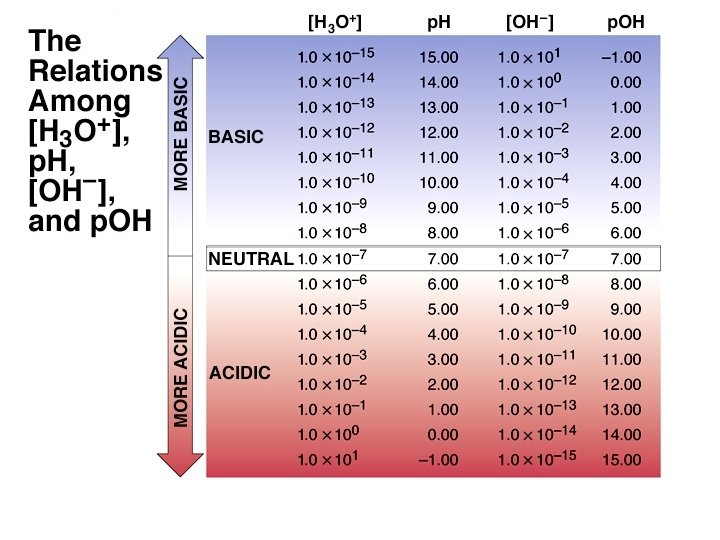The p. H Values of Some Th p. H Values of Some Familiar Aqueous Solutions (TODAY’S LAB EXPERIMENT) [H 3 O+] [OH-] = KW [H 3 O+] [OH-] [H 3 O+]> [OH-] [H 3 O+]< [OH-] acidic solution neutral solution basic solution [H 3 O+] = [OH-]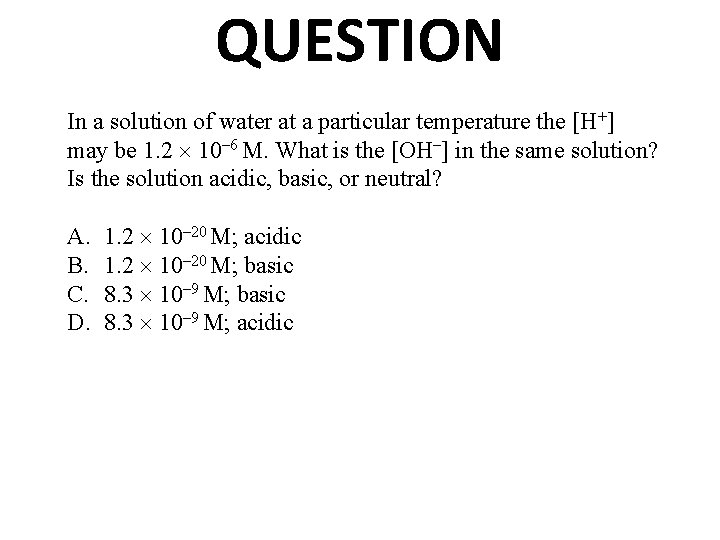QUESTION In a solution of water at a particular temperature the [H+] may be 1. 2 10– 6 M. What is the [OH–] in the same solution? Is the solution acidic, basic, or neutral? A. B. C. D. 1. 2 10– 20 M; acidic 1. 2 10– 20 M; basic 8. 3 10– 9 M; acidicANSWER D. correctly shows the OH– molarity and classifies the solution as acidic. Kw = [H+][OH–] = 1. 0 10– 14 at 25°C. The H+ molarity is approximately 1, 000 times greater than the OH– concentration. Solutions with higher H+ concentrations than OH– are acidic.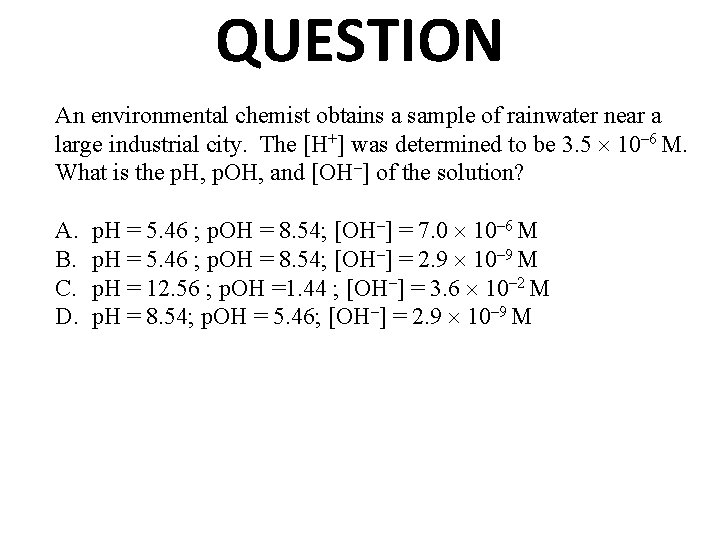QUESTION An environmental chemist obtains a sample of rainwater near a large industrial city. The [H+] was determined to be 3. 5 10– 6 M. What is the p. H, p. OH, and [OH–] of the solution? A. B. C. D. p. H = 5. 46 ; p. OH = 8. 54; [OH–] = 7. 0 10– 6 M p. H = 5. 46 ; p. OH = 8. 54; [OH–] = 2. 9 10– 9 M p. H = 12. 56 ; p. OH =1. 44 ; [OH–] = 3. 6 10– 2 M p. H = 8. 54; p. OH = 5. 46; [OH–] = 2. 9 10– 9 M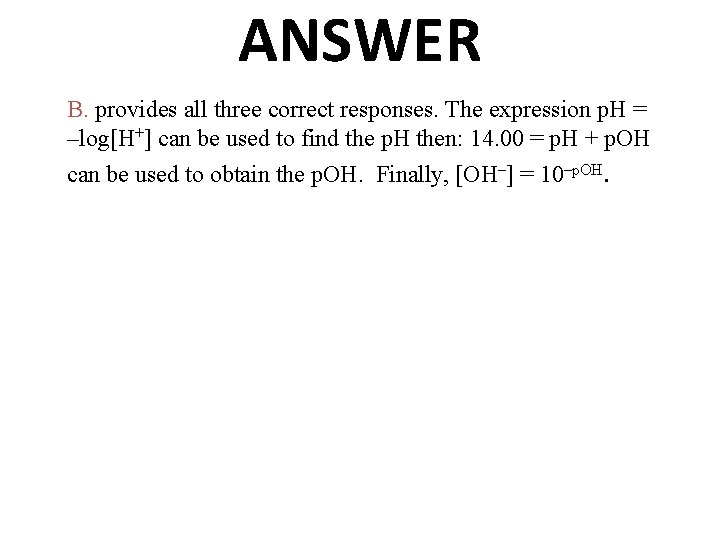ANSWER B. provides all three correct responses. The expression p. H = –log[H+] can be used to find the p. H then: 14. 00 = p. H + p. OH can be used to obtain the p. OH. Finally, [OH–] = 10–p. OH.The p. H Scale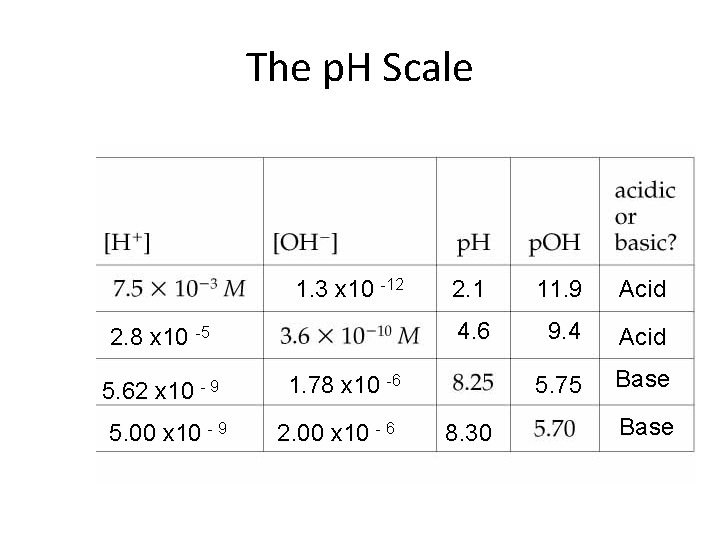The p. H Scale 1. 3 x 10 -12 2. 8 x 10 -5 5. 62 x 10 - 9 5. 00 x 10 - 9 2. 1 11. 9 Acid 4. 6 9. 4 Acid 5. 75 Base 1. 78 x 10 -6 2. 00 x 10 - 6 8. 30 Base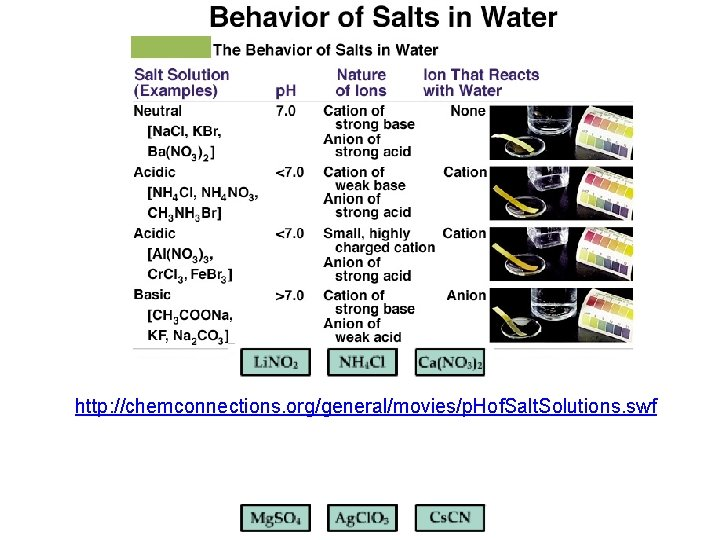http: //chemconnections. org/general/movies/p. Hof. Salt. Solutions. swf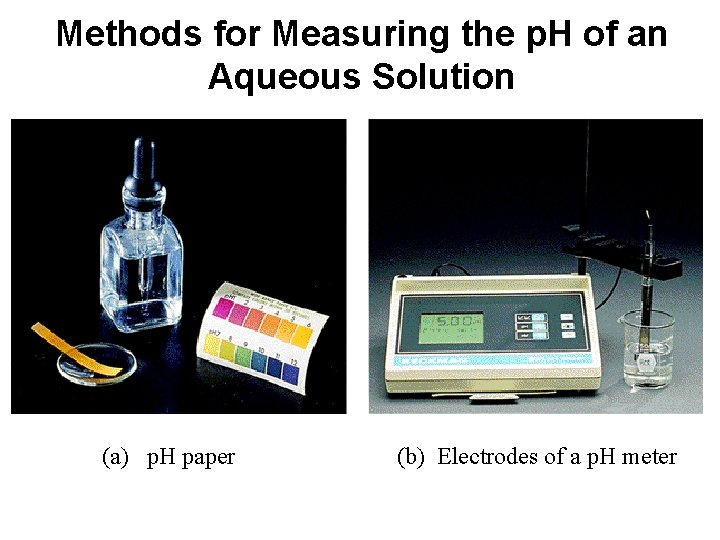Methods for Measuring the p. H of an Aqueous Solution (a) p. H paper (b) Electrodes of a p. H meterAcid-Base Equilibrium BUFFERS Dr. Ron Rusay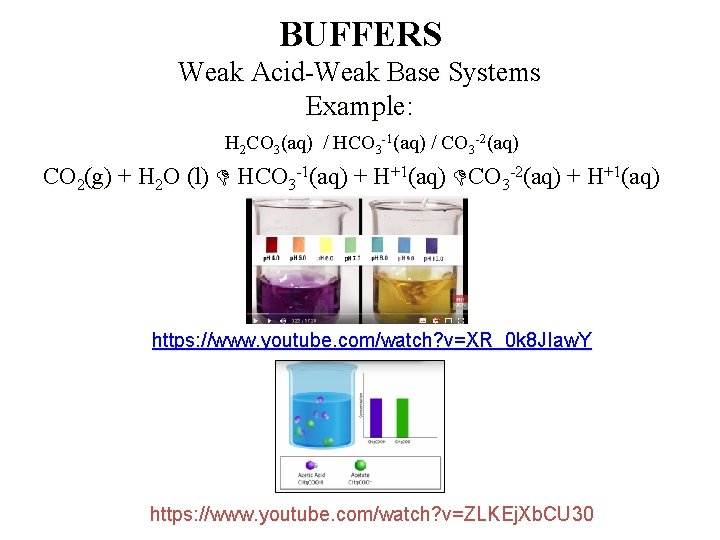BUFFERS Weak Acid-Weak Base Systems Example: H 2 CO 3(aq) / HCO 3 -1(aq) / CO 3 -2(aq) CO 2(g) + H 2 O (l) HCO 3 -1(aq) + H+1(aq) CO 3 -2(aq) + H+1(aq) https: //www. youtube. com/watch? v=XR_0 k 8 JIaw. Y https: //www. youtube. com/watch? v=ZLKEj. Xb. CU 30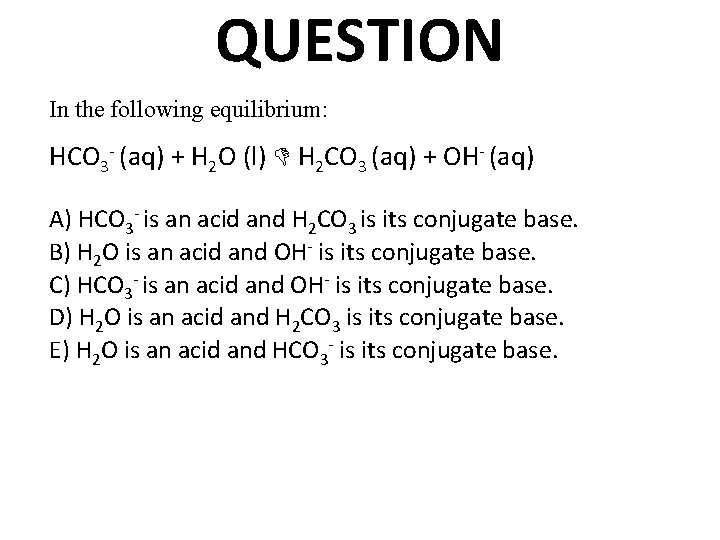QUESTION In the following equilibrium: HCO 3 - (aq) + H 2 O (l) H 2 CO 3 (aq) + OH- (aq) A) HCO 3 - is an acid and H 2 CO 3 is its conjugate base. B) H 2 O is an acid and OH- is its conjugate base. C) HCO 3 - is an acid and OH- is its conjugate base. D) H 2 O is an acid and H 2 CO 3 is its conjugate base. E) H 2 O is an acid and HCO 3 - is its conjugate base.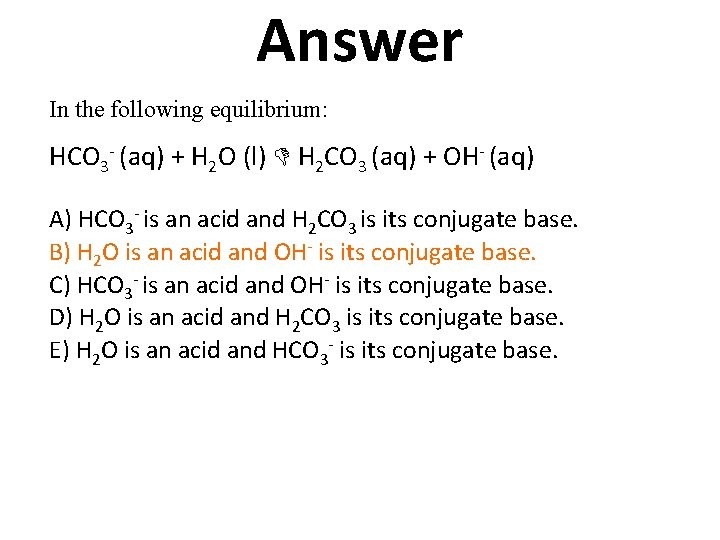Answer In the following equilibrium: HCO 3 - (aq) + H 2 O (l) H 2 CO 3 (aq) + OH- (aq) A) HCO 3 - is an acid and H 2 CO 3 is its conjugate base. B) H 2 O is an acid and OH- is its conjugate base. C) HCO 3 - is an acid and OH- is its conjugate base. D) H 2 O is an acid and H 2 CO 3 is its conjugate base. E) H 2 O is an acid and HCO 3 - is its conjugate base.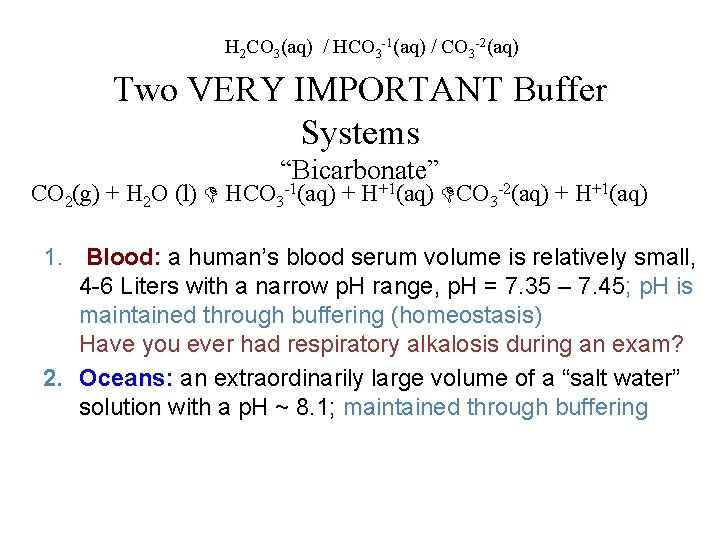H 2 CO 3(aq) / HCO 3 -1(aq) / CO 3 -2(aq) Two VERY IMPORTANT Buffer Systems “Bicarbonate” -1 +1 CO 2(g) + H 2 O (l) HCO 3 (aq) + H (aq) CO 3 -2(aq) + H+1(aq) 1. Blood: a human’s blood serum volume is relatively small, 4 -6 Liters with a narrow p. H range, p. H = 7. 35 – 7. 45; p. H is maintained through buffering (homeostasis) Have you ever had respiratory alkalosis during an exam? 2. Oceans: an extraordinarily large volume of a “salt water” solution with a p. H ~ 8. 1; maintained through buffering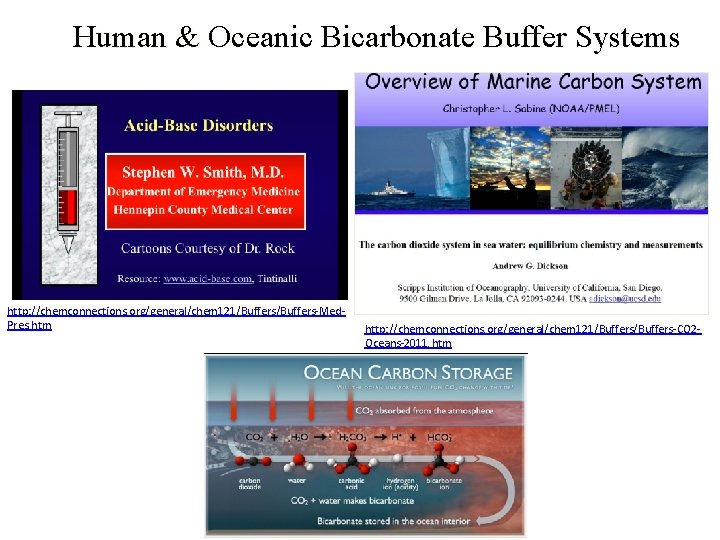Human & Oceanic Bicarbonate Buffer Systems http: //chemconnections. org/general/chem 121/Buffers-Med. Pres. htm http: //chemconnections. org/general/chem 121/Buffers-CO 2 Oceans-2011. htm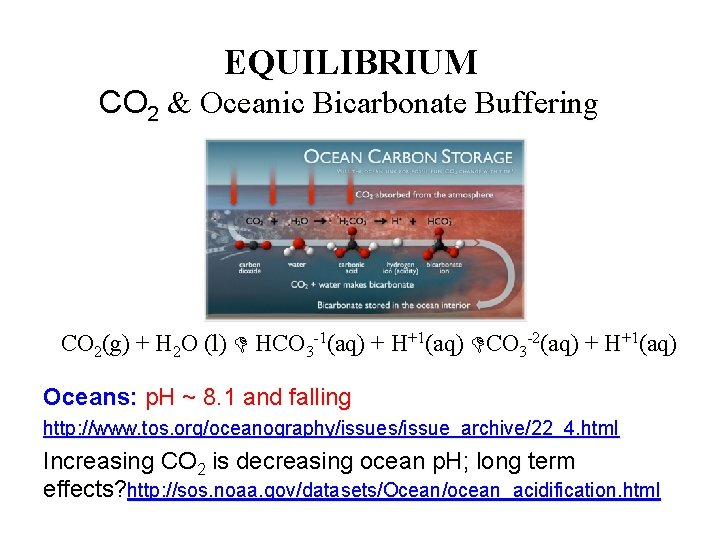EQUILIBRIUM CO 2 & Oceanic Bicarbonate Buffering CO 2(g) + H 2 O (l) HCO 3 -1(aq) + H+1(aq) CO 3 -2(aq) + H+1(aq) Oceans: p. H ~ 8. 1 and falling http: //www. tos. org/oceanography/issues/issue_archive/22_4. html Increasing CO 2 is decreasing ocean p. H; long term effects? http: //sos. noaa. gov/datasets/Ocean/ocean_acidification. html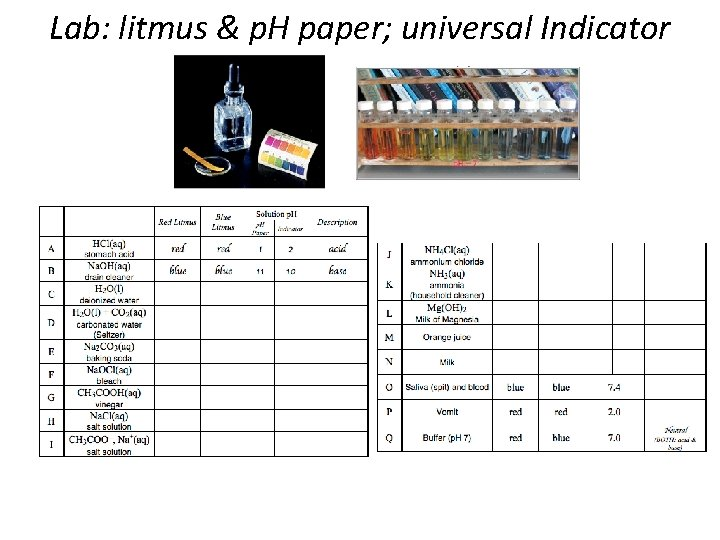Lab: litmus & p. H paper; universal IndicatorCompleted Report & Post Lab Questions Due Today: Laboratory Manual Seven Solution Problem Report Form pp. 76 -80; DUE Today POST LAB Form DUE Today http: //www. chemconnections. org/general/chem 108/7 Solutions%20 Post%20 Lab%20 form. pdf Turn in one completed form with the name of each partner who contributed on the form.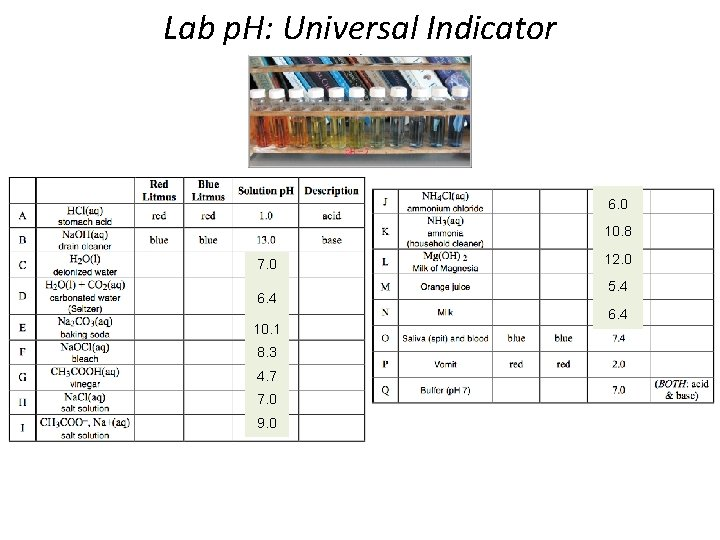Lab p. H: Universal Indicator 6. 0 10. 8 7. 0 6. 4 10. 1 8. 3 4. 7 7. 0 9. 0 12. 0 5. 4 6. 4